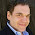## Wednesday, 18 May 2016

### PERL example for opening EXCEL sheet using WIN32:OLE

#!/usr/bin/perl
# This PERL file will open one EXCEL, create 4 WORK Sheets, in first
# WORKSheet make table with borders and few values

use Win32::OLE;
use Win32::OLE::Const 'Microsoft Excel';
\$Win32::OLE::Warn = 3;                                # die on errors...
my \$Excelfile = Win32::OLE->GetActiveObject('Excel.Application')
|| Win32::OLE->new('Excel.Application', 'Quit');
\$Excelfile->{ScreenUpdating} = 0;

for (\$j=1;\$j<=4;\$j++)
{
\$worksheet->{Name}="Summary_".\$j;
}
my \$cur_dir = `cd`;
chomp(\$cur_dir);
\$TargetFile = \$cur_dir."\\Excel_Test_File.xls";
print "Result file name is \$TargetFile \n";

#Select first sheet and write values

\$worksheet=\$workbook->WorkSheets("Summary_1");

\$current_row = 3;
\$start_row_forborder=\$current_row;
\$current_col = 2;

#for (\$k=0;\$k<3;\$k++)
#{
#
#    \$worksheet->Cells(\$current_row,\$current_col)->Font->{FontStyle}="Bold";
#    \$worksheet->Cells(\$current_row,\$current_col)->Interior->{ColorIndex} =24;
#    \$worksheet->Columns(\$current_col)->{ColumnWidth}=20;
#    \$current_col++;
#
#}

### Another way of formatting if we are using fixed columns

\$worksheet->Range("B\$current_row:D\$current_row")->Font->{FontStyle}="Bold";
\$worksheet->Range("B\$current_row:D\$current_row")->Interior->{ColorIndex} =24;
\$worksheet ->Range("B\$current_row:D\$current_row") ->{ColumnWidth} = 20;

#\$current_col=\$current_col-3;
\$worksheet->Cells(\$current_row, \$current_col)->{'Value'} = "S.No";
\$worksheet->Cells(\$current_row, \$current_col+1)->{'Value'} = "Name";
\$worksheet->Cells(\$current_row, \$current_col+2)->{'Value'} = "Department";
\$worksheet->Cells(\$current_row, \$current_col+2)->{'Value'} = "Work_type";

\$current_row++;

for (\$i=1;\$i<=10 ;\$i++) {

\$worksheet->Cells(\$current_row, \$current_col)->{'Value'} =\$i;
\$worksheet->Cells(\$current_row, \$current_col+1)->{'Value'} = "Name_".\$i;
\$worksheet->Cells(\$current_row, \$current_col+2)->{'Value'} = "Colour";
\$current_row++;
}

\$last_row=\$current_row-1;
#### Keep border for excel if it is last line.

\$worksheet->Range("B\$start_row_forborder:D\$last_row")->{HorizontalAlignment} = xlHAlignRight;
\$worksheet->Range("B\$start_row_forborder:D\$last_row")->{Borders}->{Weight} = xlThin;

#Deleting Default SHEETS
\$worksheet = \$workbook->Worksheets("Sheet1")->Delete();
\$worksheet = \$workbook->Worksheets("Sheet2")->Delete();
\$worksheet = \$workbook->Worksheets("Sheet3")->Delete();

\$workbook->Worksheets(1)->Activate;

\$workbook->SaveAs("\$TargetFile");
\$workbook->Close;

1.2.